https://doi.org/10.5194/mr-2-409-2021
Special issue:
https://doi.org/10.5194/mr-2-409-2021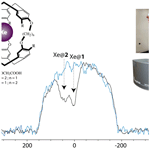# 129Xe ultra-fast Z spectroscopy enables micromolar detection of biosensors on a 1 T benchtop spectrometer

Kévin Chighine, Estelle Léonce, Céline Boutin, Hervé Desvaux, and Patrick Berthault
Abstract

The availability of a benchtop nuclear magnetic resonance (NMR) spectrometer, of low cost and easily transportable, can allow detection of low quantities of biosensors, provided that hyperpolarized species are used. Here we show that the micromolar threshold can easily be reached by employing laser-polarized xenon and cage molecules reversibly hosting it. Indirect detection of caged xenon is made via chemical exchange, using ultra-fast Z spectroscopy based on spatio-temporal encoding. On this non-dedicated low-field spectrometer, several ideas are proposed to improve the signal.

Share
Dates
1 Introduction

In this sad period overshadowed by pandemic, among the analytical methods aiming at imaging the lung–blood transfer, hyperpolarized xenon nuclear magnetic resonance/magnetic resonance imaging (NMR/MRI) increasingly interests the “in vivo” scientific community. While xenon nuclear polarization can easily be boosted via spin-exchange optical pumping (Walker and Happer1997, SEOP), the other interest of this noble gas for NMR is that it exhibits a wide chemical shift range (more than 320 ppm for the monoatomic species) and is soluble in most biological fluids. Therefore, xenon is a powerful exogenous probe of the functioning of the air–blood barrier . Moreover, it is prone to opening the way to molecular magnetic resonance imaging. In an approach pioneered by A. Pines and co-workers , xenon is reversibly encapsulated in molecular systems that are functionalized with biological ligands. This two-step procedure, where the bioprobe is first introduced and hyperpolarized xenon then delivered, benefits from the difference in resonance frequency between bound xenon and free xenon (in the gas phase or in the dissolved phase; cf. ).

In this method, most of the studies used cryptophane derivatives as xenon hosts, as despite a complex synthesis they are functionalizable by ligands . For instance, to our knowledge, only one example of chemical functionalization of a xenon host other than cryptophane – a cucurbituril – has been reported in the literature . The approach of 129Xe NMR-based biosensing using functionalized cryptophanes has been successfully applied in vitro for detection of small analytes , of large biosystems , or of change in physiological conditions: temperature or pH . To date, it has however never been used in vivo; only a proof of concept has been performed on rats using a non-functionalized cucurbituril . Several difficulties or obstacles have delayed in vivo applications, among which is obviously the lack of sensitivity.

We made the remark that in a pre-clinical environment, it could be very useful to test the behavior of such bioprobes in NMR, using a benchtop spectrometer, less cumbersome and less expensive than a high-field spectrometer. It could be placed very close to the optical pumping setup, working in flow or batch modes. The present work aims at assessing the feasibility of the detection of low concentrations of 129Xe NMR-based biosensors using a non-dedicated benchtop spectrometer. After a brief description of our spin-exchange optical pumping setup working in the batch mode, direct and indirect detection techniques are studied at both low and high magnetic fields. Theoretical considerations are given, and practical ways of improvement are analyzed.

2 Results

## 2.1 Molecular systems

For such a study, we decided to use two water-soluble cryptophanes, synthesized by the group of T. Brotin at ENS Lyon and previously characterized . Their generic structure is depicted in Fig. 1. In cryptophane 1 the two cyclotriveratrilene bowls are connected by two O-(CH2)2-O linkers and a O-(CH2)3-O linker; in cryptophane 2 they are connected by two O-(CH2)3-O linkers and a O-(CH2)2-O linker. Xenon inside these cage molecules resonates at 52 ppm (for Xe@1) and 42 ppm (for Xe@2) if one calibrates the signal of free xenon in water at 196 ppm. These two molecular systems could be used as probes for pH, as the chemical shift of caged xenon varies as a function of the concentration in H+ ions .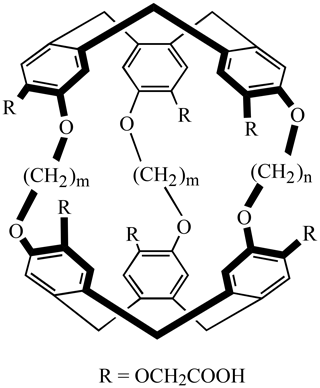Figure 1Generic structure of the cryptophanes used in this study. For compound 1: m=2 and n=3; for 2: m=3 and n=2.

## 2.2 Addition of hyperpolarized xenon into the samples

The principle of the experiments is depicted in Fig. 2. Xenon enriched at 83 % in isotope 129 was polarized via spin-exchange optical pumping using a home-made setup already described in . After about 10 min of optical pumping, hyperpolarized xenon was collected frozen and transported immersed in liquid nitrogen inside a 0.3 T solenoid fed by a car battery. Then, in the fringe field of the unshielded 11.7 T magnet, xenon was heated and transferred to the capped NMR tube containing the sample, thanks to a vacuum line and a hollow spinner filled with liquid nitrogen. This procedure enabled us to transfer all xenon above the solution without freezing it. Finally, fast heating and vigorous shaking of the tube sped up the dissolution of the noble gas into the sample of interest. This method, proven efficient for high magnetic field experiments, is not directly translatable to the NMR experiments in the benchtop spectrometer. All attempts to use the fringe field of the permanent magnet during the xenon transfer and shaking step led to disappointing results in terms of remaining polarization. This was expected as the magnet, of the Halbach type, delivers a horizontal static magnetic field with a steep slope in intensity and direction in the vertical dimension, and thus xenon may cross areas of a null field during its introduction in the spectrometer. The least bad solution was thus to carry out the introduction of polarized xenon inside the NMR tube in the fringe field of the superconducting magnet and then to quickly shake the tube in this magnetic environment before transporting it to the benchtop spectrometer. The following shaking, carried out as close as possible to the permanent magnet, leads to a faster depolarization.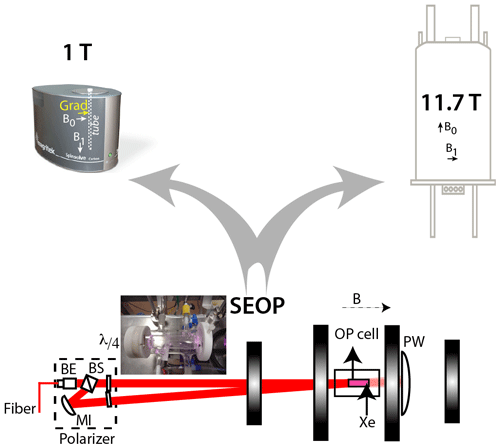Figure 2Principle of the experiments. Laser-polarized xenon is produced via spin-exchange optical pumping in batch mode with the setup described in . BE: beam expander; BS: polarization beam-splitter cube; MI: mirror; $\mathit{\lambda }/\mathrm{4}$: quarter-wave plates; PW: power meter. The black vertical rectangle represents the coils, which deliver a magnetic field B co-linearly to the photon beam. A picture of the optical pumping cell is given in the inset. After some minutes of optical pumping, frozen hyperpolarized xenon is transported to the NMR spectrometers. The disposition of the NMR tube inside the magnet, the static and radiofrequency fields as well as the axis of the gradient are indicated on the benchtop spectrometer.

Without optimization other than the setting of the quarter-wave plates, this robust setup gave us a useable polarization of ca. 0.15, as measured in the gas phase on the 11.7 T spectrometer by comparison with the thermal equilibrium NMR signal.

The pressure in the NMR tube on top of the solution was ca. 1 bar, as estimated post NMR experiment by weighing the tube before and after degassing.

## 2.3 Direct detection of 129Xe NMR-based biosensors

Figure 3 displays a comparison of one-scan 129Xe NMR spectra of the same sample of cryptophanes dissolved at a concentration of 77 µM in D2O, at 11.7 and 1 T (same NMR tube). The two spectra were recorded with different xenon batches but acquired in the same experimental conditions.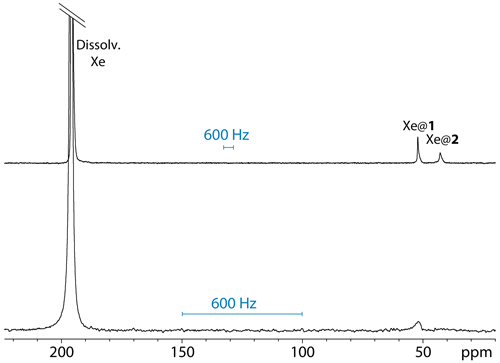Figure 3One-scan 129Xe NMR spectra of the noble gas into a water solution of cryptophanes 1 and 2, both at 77 µM and 295 K, with the same pulse flip angle. Top spectrum performed at 11.7 T; bottom spectrum performed at 1 T.

Not surprisingly, the signal-to-noise ratio is better at 11.7 T than at 1 T. For information purposes only, they were measured at 2492 and 1142, respectively, taking into account the signal of free xenon in water. Obviously, if the xenon nuclear polarization should be similar for the two experiments as it directly derives from the optical pumping step, the signal-to-noise ratios are expected to be very different due to the detection part. Indeed, in classical NMR, it is the magnetic induction proportional to the temporal derivative of the component of the magnetization perpendicular to the static magnetic field that is detected, and thus the Larmor frequency on the one hand and all geometrical and electronic parameters defining the sensitivity (coil geometry, useful volume, quality factor, tuning frequency, filter and digitization) on the other hand would have to be considered for comparing the signal-to-noise ratio.

From Fig. 3, several remarks can be made. While at high field the 129Xe NMR spectrum displays one signal at 196 ppm corresponding to free xenon in water and two distinct signals at high field corresponding to caged xenon (Xe@1 at 52 ppm and Xe@2 at 42 ppm according to ), at low static magnetic field one of the latter signals disappears or is hardly observable. The full-width at half-maximum of the Xe@1 signals was roughly measured to 44 and 20 Hz at 11.7 and 1 T, respectively. The presence of a narrow peak at 11.7 T and a very broad line at 1 T for Xe@2 reveals the weakness of the direct detection approach, even if one uses a fast repetition “frequency-selective pulse – detection” . When the lines are so broad and the signal to noise so low, each of these acquisitions brings a lot of noise, and the resulting signal is difficult to distinguish from the baseline.

## 2.4 Indirect detection

It is well known that the detection of diluted species in exchange with a main spin reservoir can be facilitated by taking advantage of this exchange. This has given rise to the Chemical Exchange Saturation Transfer (CEST) experiments, whose principle lies in the radio-frequency (rf) saturation at the frequency of these diluted species and the observation of the subsequent loss of magnetization of the main reservoir due to the exchange (see for instance ). Schröder and co-workers have extended this approach to hyperpolarized species, creating the Hyper-CEST sequence .

However, sequences of the CEST family are usually restricted to high magnetic fields. For instance, for detection of metabolites such as glutamate or carnosine, 1H CEST is an efficient method . However, it would not come to mind to use a CEST sequence with a low magnetic field, as the frequency splitting between the two environments in exchange is only ca. 3.3 ppm (140 Hz at 1 T). However, due to the very wide chemical shift range of xenon, this can be envisioned in the case of 129Xe NMR-based biosensors.

Due to the huge advantage of a spectrum-per-spectrum averaging instead of a point-per-point averaging for hyperpolarized species, we decided to turn to the ultra-fast version of the CEST experiment, initially proposed by Jerschow and co-workers in 1H . In this sequence, saturation is applied in the presence of a gradient, which amounts to saturating only a slice of the sample. After a read pulse, the receiver is open in the presence of a second gradient which decodes the profile of the sample along it. By subtracting two experiments recorded with and without saturation, one obtains the Z spectrum. Note that this sequence can be combined with localized spectroscopy or imaging schemes; see, for instance, , , and .

In the past, the ultra-fast Z spectroscopy (UFZ) experiment was successfully applied in hyperpolarized 129Xe NMR for the study of biosensors by us and others and also for detection of low numbers of biological cells .

Note that Gouilleux and co-workers were the first to use pulsed field gradients and to implement spatially encoded pulse sequences on a low magnetic field spectrometer, which allowed access to experiments such as DOSY and ultra-fast COSY; see a review in . On the other hand, King et al. have demonstrated the performance of ultra-fast multidimensional Laplace NMR – based also on spatial encoding – on a low-field, single-sided magnet to derive T1T2 and D–T2 correlation maps . This method reveals its full potential when used with DNP hyperpolarized species.

For this study, we used the pulse sequence depicted in Fig. 4a. It contains an rf offset switch: the first value, ωB, is centered on the Xe@cryptophanes region (i.e., around 45 ppm), while the second value, ωA, is applied on-resonance with the free xenon frequency. Such a sequence is not intended to provide the full Z spectrum (saturation at the free xenon frequency would be disastrous for hyperpolarization) but is prone to revealing the presence of caged xenon (cf. , for details).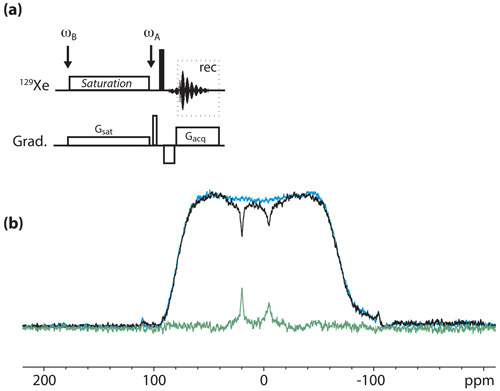Figure 4(a) Pulse sequence used for the 129Xe UFZ spectroscopy. ωB and ωA denote the rf offset placed in the region of caged xenon and at the frequency of free xenon, respectively. (b) 129Xe UFZ spectrum of the noble gas into the solution containing the cryptophane mixture at 11.7 T and 295 K. In black: the profile obtained after 2 s saturation at a field strength ${B}_{\mathrm{1}}={\mathit{\omega }}_{\mathrm{1}}/\mathit{\gamma }=\mathrm{7}$µT (on experiment); in blue: same profile without saturation (off experiment); in green: off–on. Other important parameters: Gsat=35 mT m−1; Gacq=90 mT m−1.

Figure 4b displays an 129Xe UFZ spectrum recorded on the cryptophane mixture at 11.7 T and 295 K.

The apparent chemical shift splitting between the two dips can be measured at 25.4 ppm, which corresponds well to the real chemical shift splitting of 10 ppm when the ratio between the saturation and acquisition gradients is considered (see the theoretical part). The dip corresponding to Xe@2 is broader than that of Xe@1, which can be explained by a faster xenon in–out exchange.

Figure 5 displays an 129Xe UFZ spectrum recorded on the cryptophane mixture at 1 T and 295 K. For the spatial encoding of the 129Xe UFZ-spectroscopy experiment, on the Magritek SpinSolve C spectrometer we had the choice of using the shim system to create pulsed field gradients in the x, y or z directions or using the installed gradient system. The latter gradient is oriented horizontally, while the main axis of the NMR tube is vertical (see Fig. 2). It could have been interesting to use the shim along the NMR tube axis, as the magnetization profile would have been along the largest dimension of the tube and would have given a flat profile. However, for stability reasons we decided to use the nominal gradient system. This explains the rounded shape of the magnetization profile envelope, corresponding to an axial projection of the tube. Note that quantitative interpretation of the UFZ spectra, e.g., to extract exchange rates from a set of UFZ spectra, would need consideration of the excitation–detection profile of the rf coil .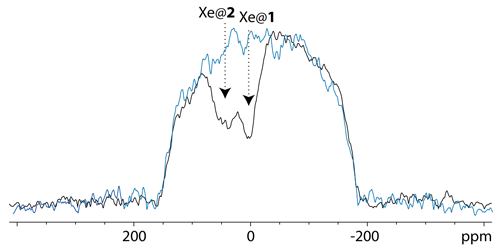Figure 5129Xe UFZ spectrum of the noble gas into the solution containing the cryptophane mixture at 1 T and 295 K, using the same pulse sequence as for Fig. 4 (sum of two experiments with saturations of 2 and 4 s). The saturation offset was placed at the Xe@1 frequency. In black: the profile obtained after saturation at a field strength ${B}_{\mathrm{1}}={\mathit{\omega }}_{\mathrm{1}}/\mathit{\gamma }=$ 0.87 µT (on experiment); in blue: same profile without saturation (off experiment); other important parameters: Gsat=21 mT m−1; Gacq= 84 mT m−1.

The gradient strength was firstly calibrated by acquiring a 1D axial profile of the tube in 1H. Then we chose the value of the gradient simultaneous to 129Xe saturation, Gsat, keeping in mind that it had to induce a spectral expansion of the signal saturation smaller than the difference between the free xenon frequency ωA and the caged xenon frequency ωB, thus filling the condition

$\mathit{\gamma }\cdot {G}_{\text{sat}}\cdot d<|{\mathit{\omega }}_{\mathrm{A}}-{\mathit{\omega }}_{\mathrm{B}}|,$

with d the inner diameter of the tube. In our case, d=0.43 cm, $\mathit{\gamma }=-\mathrm{11.79}$ MHz T−1, and $|{\mathit{\omega }}_{\mathrm{A}}-{\mathit{\omega }}_{\mathrm{B}}|\simeq \mathrm{1800}$ Hz means that Gsat must be lower than 35.5 mT m−1.

The profile of the UFZ spectrum reveals two dips corresponding to xenon in the two cryptophanes, with an apparent frequency splitting of about 40 ppm, which is the expected value for Xe@1 and Xe@2 given the ratio ${G}_{\text{sat}}/{G}_{\text{acq}}$. The rf carrier having been placed on resonance with the Xe@1 frequency, we have assigned the dips as displayed in Fig. 5. The apparent reverse frequency axis is due to the relative sign of the two gradients. Obviously the separation between the dips is less net than at high field, and to confirm our assignment, we have performed the same experiment with another xenon batch, simply inverting the sign of Gsat. The corresponding two-scan UFZ spectrum is displayed in Fig. 6.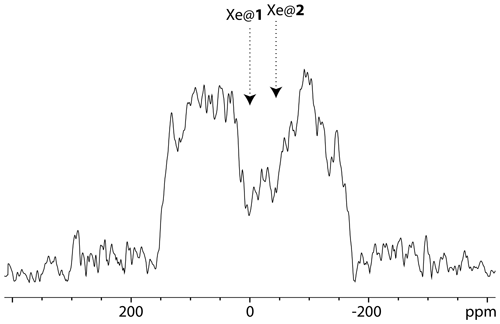Figure 6129Xe UFZ spectrum of the noble gas into the solution containing the cryptophane mixture at 1 T and 295 K, using the same pulse sequence as for Fig. 5, except that the saturation gradient is opposite: ${G}_{\text{sat}}=-\mathrm{21}$ mT m−1; Gacq=84 mT m−1. Saturation delay: 3 s.

In order to model the behavior of the Z spectra as a function of the experimental parameters, we have simulated the Hyper-CEST experiment (see theoretical section). As input of these simulations, ${R}_{{\mathrm{1}}_{\mathrm{A}}}$ can be measured in a experiment consisting of a series {small flip angle pulse – detection} by taking into account the flip angle. ${R}_{{\mathrm{2}}_{\mathrm{A}}}$ can be roughly estimated from the line width of A but with a large uncertainty. f can be directly determined from a simple 129Xe spectrum as it is the ratio of signal B to signal A. However, the parameter which remains difficult to predict or estimate is kout.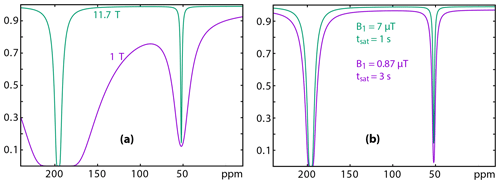Figure 7Simulation of 129Xe Z spectra at 11.7 (green) and 1 (purple) Tesla. (a) Considering the same relaxation times, same rf field strength and duration of the saturation; (b) considering a saturation of lower amplitude but larger duration for the low-field experiment. Details of the conditions for the simulation: see text.

In a first step, we used Eqs. (13) and (11) to simulate the trends on the 129Xe UFZ spectra recorded at 11.7 and 1 T (Fig. 7). In Fig. 7a, in order to show the effect of the Larmor frequency, all other parameters have been kept identical (f is the fraction of caged xenon; kout is the xenon out rate): f=0.04; kout=50 s−1; δA=196 ppm; δB=52 ppm; ${R}_{{\mathrm{1}}_{\mathrm{A}}}=\mathrm{0.01}$ s−1; ${R}_{{\mathrm{2}}_{\mathrm{A}}}=\mathrm{50}$ s−1; ω1=521.4 rad s−1 (7 µT); tsat=1 s.

This simulation shows that the rf saturation strength must be reduced on the low-field spectrometer in order to keep a flat baseline, due to the low difference between the free and bound xenon resonance frequencies.

Figure 7b displays the simulation for more realistic experimental conditions. The parameters were the following.

• Parameters common to both fields: f=0.04; kout=50 s−1; δA=196 ppm; δB=52 ppm

• Spinsolve (Larmor frequency = 12.09 MHz): ${R}_{{\mathrm{1}}_{\mathrm{A}}}=\mathrm{0.01}$ s−1; ${R}_{{\mathrm{2}}_{\mathrm{A}}}=\mathrm{20}$ s−1; ω1=64.7 rad s−1 (0.87 µT); tsat=3 s

• Avance (Larmor frequency = 138.36 MHz): ${R}_{{\mathrm{1}}_{\mathrm{A}}}=\mathrm{0.01}$ s−1; ${R}_{{\mathrm{2}}_{\mathrm{A}}}=\mathrm{50}$ s−1; ω1=521.4 rad s−1 (7 µT); tsat=1 s

Comparison between the two 129Xe Z spectra simulated with realistic conditions evidences firstly the effect of the smaller frequency splitting on Magritek. Despite lower relaxation rates and despite a weaker saturation strength at low field, the direct relaxation term λdirect(ωi,ω1) has a larger influence on the baseline in the Xe@cryptophane region (purple curve). This term decreases the signal by about 3 %. This reveals that the saturation field strength must stay small to ensure the success of the experiment. Secondly, such a small ω1 requires a longer saturation time. With a saturation time tsat 3 times longer than the one used at high field, the dip in the Xe@cryptophane region has almost the same depth.

The second simulation considered two cryptophanes with characteristics close to the ones used in the present study. The parameters of the 129Xe Z spectra were those of the real experiment on the benchtop spectrometer:

• Parameters common to both cryptophanes: Larmor frequency = 12.09 MHz; ${R}_{{\mathrm{1}}_{\mathrm{A}}}=\mathrm{0.1}$ s−1; ${R}_{{\mathrm{2}}_{\mathrm{A}}}=\mathrm{10}$ s−1; tsat=3 s

• Cryptophane 1: f=0.037; kout=50 s−1; δB=52 ppm

• Cryptophane 2: f=0.025; kout=100 s−1; δB=42 ppm

Figure 8 displays the effect of the saturation strength ω1 on the aspect of the 129Xe Z spectrum of the cryptophane mixture (only the 0–100 ppm region is displayed). At low saturation strength, the signal of the cryptophane with the highest $f/{k}_{\text{out}}$ is predominant, as expected (see theoretical part). Then, when ω1 increases, the second dip becomes more pronounced, larger than the first one due to the higher kout value (Eq. 9). However, increasing further ω1 leads to significant lowering of the baseline and thus of the contrast, and the signals are less separated. Thus a compromise has to be found to favor detection of the dips and maximize their separation. With the current conditions of the simulation, a value of ω1 between 50 and 150 rad s−1 seems best.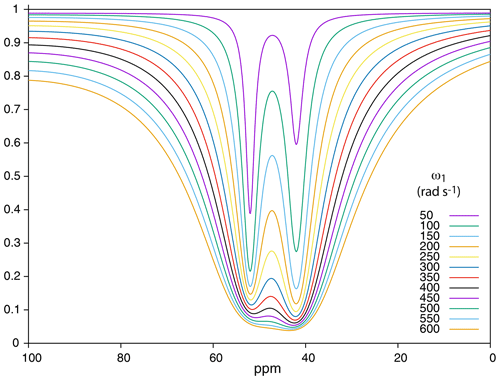Figure 8Simulation of 129Xe Z spectra at 1 T for a mixture of two cryptophanes according to the saturation field strength. Conditions for the simulation: see text.

These simulations have helped us to find the best experimental conditions.

In such an approach, the detection threshold can further be lowered, maybe at the price of a lack of discrimination between two minor sites in exchange with the main signal. As an example that does not seek to be a record, this experiment has been repeated with a solution containing cryptophane 2 at a concentration of 19.2 µM. Figure 9 displays the 129Xe UFZ profile. With respect to the previous experiments, only the saturation field strength has been slightly increased from 0.87 to 1.24 µT (ω1=64.7 to 92.4 rad s−1). The dip corresponding to caged xenon appears clearly.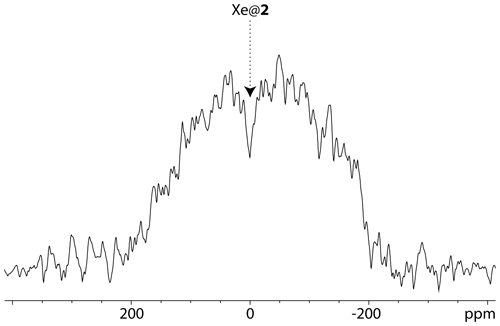Figure 9129Xe UFZ spectrum of the noble gas into the solution containing cryptophane 2 at 19.2 µM. Magnetic field: 1 T; temperature 295 K, same pulse sequence as for Fig. 4 (one scan). ωB was placed at the Xe@2 frequency. Profile obtained after 3 s saturation at a field strength ${B}_{\mathrm{1}}={\mathit{\omega }}_{\mathrm{1}}/\mathit{\gamma }=\mathrm{1.24}$µT. Gsat=21 mT m−1; Gacq=84 mT m−1.

3 Materials and methods

## 3.1 Preparation of the samples

A mother solution of cryptophane 1 was prepared by dissolution of 0.64 mg of the powder in 500 µL D2O and 20 µL NaOD 0.1 M. The same preparation was done for cryptophane 2; 50 µL of each of these solutions were mixed, and then the solution was diluted by a factor 5 in D2O, giving resulting concentrations of 77 µM for cryptophane 1 and 78 µM for cryptophane 2. The solution was placed in a 5 mm NMR tube equipped with a valve. The tube was degassed before each introduction of hyperpolarized xenon by three cycles of helium bubbling and then evacuation.

## 3.2 Observation of 129Xe on the 11.7 T spectrometer

The experiments were performed at 295 K on a Bruker Avance II spectrometer equipped with a dual 129Xe-1H probe head and a GREAT3/10 three-axis gradient amplifier.

## 3.3 Observation of 129Xe on the benchtop spectrometer

The experiments were performed at 295 K on a Magritek SpinSolve Carbon spectrometer at a magnetic field of 1.02 T (1H Larmor frequency 43.71 MHz), the temperature of the permanent magnet being 301 K. The X channel of the low-field spectrometer being initially optimized for 13C (i.e., 10.9 MHz), it was necessary to lower the temperature of the permanent magnet in order to approach the 129Xe Larmor frequency (12.1 MHz). Obviously, the situation is not optimal for 129Xe, and the value of the 90 pulse was measured to 150 µs at full power. This was calibrated with a sample of thermal xenon inside dodecane doped with Gd3+ ions. Note that at such a low field, the paramagnetic doping was not very efficient, and a T1 of ca. 10 s was found for 129Xe in this solution. The same procedure was used to calibrate the B1 strength value for saturation.

The SpinSolve software in expert mode was used to create the pulse programs that were not in the Magritek library. In particular, the ultra-fast Z spectroscopy sequence was written and tested, first in 1H NMR and then in 23Na NMR, before the 129Xe NMR experiments. The pulse program written for the Magritek Spinsolve spectrometer is provided in the Supplement.

In order to apply exactly the same processing to the data acquired at 1 and 11.7 T and to compare them safely, a program was written in Python to convert the FID recorded under Spinsolve into JCAMP-DX data readable by the Bruker software, Topspin.

4 Theory of the Hyper-CEST experiment

Let us consider a cryptophane solubilized in water at a sub-millimolar concentration. A small part of hyperpolarized xenon, when introduced in solution, will be reversibly caged in cryptophane. The 129Xe spectrum will thus exhibit two signals: free dissolved xenon (pool A of magnetization ${M}_{\mathrm{A}}^{\mathrm{0}}$, giving rise to the main signal at ωA) and xenon inside the cryptophane (pool B of magnetization ${M}_{\mathrm{B}}^{\mathrm{0}}$, very minor signal at ωB). Two types of xenon in–out exchange coexist: simple dissociation,

$\begin{array}{}\text{(1)}& X+C\underset{{k}_{-}}{\overset{{k}_{+}}{⇌}}CX,\end{array}$

(X for xenon, C for cryptophane), and degenerate (or kick-out) exchange, dependent on the ratio xenon concentration to cryptophane concentration :

$\begin{array}{}\text{(2)}& CX+{X}^{\ast }\underset{k}{⇌}C{X}^{\ast }+X,\end{array}$

where the asterisk denotes the hyperpolarized state. As the two xenon pools have different resonance frequencies, these processes can be seen as a unique exchange between free and encapsulated xenon, characterized by the rates kin and kout at steady state:

$\begin{array}{}\text{(3)}& {k}_{\text{in}}={k}_{\text{out}}\frac{{M}_{\mathrm{B}}^{\mathrm{0}}}{{M}_{\mathrm{A}}^{\mathrm{0}}}={k}_{\text{out}}×f,\end{array}$

recalling that f is the fraction of caged xenon.

The Hyper-CEST experiment consists in saturating with a (CW) rf irradiation of strength ω1 in the Xe@cryptophane region (pool B) and detecting the influence on the main Xe signal (pool A). This has two consequences.

• A direct effect linked to the rf saturation at an offset ${\mathrm{\Delta }}_{i}={\mathit{\omega }}_{i}-{\mathit{\omega }}_{\mathrm{A}}$ from the main resonance, which tilts the magnetization by an angle ${\mathit{\theta }}_{i}={\mathrm{tan}}^{-\mathrm{1}}\frac{{\mathit{\omega }}_{\mathrm{1}}}{|{\mathrm{\Delta }}_{i}|}$ with θi in $\left[-\mathit{\pi }/\mathrm{2}:\mathit{\pi }/\mathrm{2}\right]$ . The magnetization transverse to this effective field is averaged out by rf field inhomogeneity and transverse relaxation. The magnetization aligned with the effective field is going to relax. This effect, present even in the absence of exchange, is characterized by the depolarization rate:

$\begin{array}{}\text{(4)}& {\mathit{\lambda }}_{\text{direct}}\left({\mathit{\omega }}_{i},{\mathit{\omega }}_{\mathrm{1}}\right)={R}_{{\mathrm{1}}_{\mathrm{A}}}{\mathrm{cos}}^{\mathrm{2}}{\mathit{\theta }}_{i}+{R}_{{\mathrm{2}}_{\mathrm{A}}}{\mathrm{sin}}^{\mathrm{2}}{\mathit{\theta }}_{i},\end{array}$

where ${R}_{{\mathrm{1}}_{\mathrm{A}}}$ and ${R}_{{\mathrm{2}}_{\mathrm{A}}}$ are the longitudinal and transverse relaxation rates of free xenon, respectively. In the range of chemical exchange observed with cryptophanes $k={k}_{\text{in}}+{k}_{\text{out}}\gg {R}_{{\mathrm{1}}_{\mathrm{A}}},{R}_{{\mathrm{2}}_{\mathrm{A}}}$, given the low proportion of xenon caged in the cryptophane, one has

$\begin{array}{}\text{(5)}& {R}_{\mathrm{1}}=\left(\mathrm{1}-f\right){R}_{{\mathrm{1}}_{\mathrm{A}}}+f{R}_{{\mathrm{1}}_{\mathrm{B}}}\simeq {R}_{{\mathrm{1}}_{\mathrm{A}}},\text{(6)}& {R}_{\mathrm{2}}=\left(\mathrm{1}-f\right){R}_{{\mathrm{2}}_{\mathrm{A}}}+f{R}_{{\mathrm{2}}_{\mathrm{B}}}\simeq {R}_{{\mathrm{2}}_{\mathrm{A}}}.\end{array}$
• An indirect depolarization due to the saturation transfer from pool B to pool A (CEST effect). If the saturation is applied exactly on-resonance with the caged xenon frequency (ωi=ωB), with the assumption ${k}_{\text{out}}\gg {R}_{\mathrm{2}}^{B}$ and koutkin, the depolarization rate linked to saturation at the frequency of pool B is given by

$\begin{array}{}\text{(7)}& {\mathit{\lambda }}_{\text{on}}\left({\mathit{\omega }}_{\mathrm{1}}\right)\simeq f{k}_{\text{out}}\frac{{\mathit{\omega }}_{\mathrm{1}}^{\mathrm{2}}}{{\mathit{\omega }}_{\mathrm{1}}^{\mathrm{2}}+{k}_{\text{out}}^{\mathrm{2}}}.\end{array}$

This has enabled Kunth and co-workers to distinguish three cases :

• ${\mathit{\omega }}_{\mathrm{1}}\gg f{k}_{\text{out}}:{\mathit{\lambda }}_{\text{on}}\simeq f{k}_{\text{out}}$. Maximum depolarization rate.

• ${\mathit{\omega }}_{\mathrm{1}}=f{k}_{\text{out}}:{\mathit{\lambda }}_{\text{on}}\simeq f{k}_{\text{out}}/\mathrm{2}$. Fifty percent of the maximum possible depolarization rate.

• ${\mathit{\omega }}_{\mathrm{1}}\ll f{k}_{\text{out}}:{\mathit{\lambda }}_{\text{on}}\simeq \left(f/{k}_{\text{out}}\right){\mathit{\omega }}_{\mathrm{1}}^{\mathrm{2}}$. Parabolic behavior with the saturation strength.

In order to understand the UFZ-spectroscopy experiment, one needs to consider the difference frequency between the frequency of the rf irradiation, ωi, and the frequency of pool B, ωB, which has a Lorentzian shape as a function of ωi .

$\begin{array}{}\text{(8)}& {\mathit{\lambda }}_{\text{CEST}}\left({\mathit{\omega }}_{i},{\mathit{\omega }}_{\mathrm{1}}\right)=\frac{{\mathit{\lambda }}_{\text{on}}\left({\mathit{\omega }}_{\mathrm{1}}\right)\frac{\mathrm{\Gamma }\left({\mathit{\omega }}_{\mathrm{1}}{\right)}^{\mathrm{2}}}{\mathrm{4}}}{\frac{\mathrm{\Gamma }\left({\mathit{\omega }}_{\mathrm{1}}{\right)}^{\mathrm{2}}}{\mathrm{4}}+\left({\mathit{\omega }}_{i}-{\mathit{\omega }}_{\mathrm{B}}{\right)}^{\mathrm{2}}},\end{array}$

with the full-width at half-maximum value of the depolarization rate given by

$\begin{array}{}\text{(9)}& \mathrm{\Gamma }\left({\mathit{\omega }}_{\mathrm{1}}\right)\simeq \mathrm{2}\sqrt{{\mathit{\omega }}_{\mathrm{1}}^{\mathrm{2}}+{k}_{\text{out}}^{\mathrm{2}}}.\end{array}$

Thus, the total depolarization rate is

$\begin{array}{}\text{(10)}& \begin{array}{rl}\mathit{\lambda }\left({\mathit{\omega }}_{i},{\mathit{\omega }}_{\mathrm{1}}\right)& ={\mathit{\lambda }}_{\text{direct}}\left({\mathit{\omega }}_{i},{\mathit{\omega }}_{\mathrm{1}}\right)+{\mathit{\lambda }}_{\text{CEST}}\left({\mathit{\omega }}_{i},{\mathit{\omega }}_{\mathrm{1}}\right)\\ & ={R}_{{\mathrm{1}}_{\mathrm{A}}}{\mathrm{cos}}^{\mathrm{2}}{\mathit{\theta }}_{i}+{R}_{{\mathrm{2}}_{\mathrm{A}}}{\mathrm{sin}}^{\mathrm{2}}{\mathit{\theta }}_{i}\\ & +\frac{{\mathit{\lambda }}_{\text{on}}\left({\mathit{\omega }}_{\mathrm{1}}\right)\frac{\mathrm{\Gamma }\left({\mathit{\omega }}_{\mathrm{1}}{\right)}^{\mathrm{2}}}{\mathrm{4}}}{\frac{\mathrm{\Gamma }\left({\mathit{\omega }}_{\mathrm{1}}{\right)}^{\mathrm{2}}}{\mathrm{4}}+\left({\mathit{\omega }}_{i}-{\mathit{\omega }}_{\mathrm{B}}{\right)}^{\mathrm{2}}}.\end{array}\end{array}$

which can be re-written as

$\begin{array}{}\text{(11)}& \begin{array}{rl}\mathit{\lambda }\left({\mathit{\omega }}_{i},{\mathit{\omega }}_{\mathrm{1}}\right)& ={R}_{{\mathrm{1}}_{\mathrm{A}}}\frac{\mathrm{1}}{\mathrm{1}+{\left(\frac{{\mathit{\omega }}_{\mathrm{1}}}{{\mathit{\omega }}_{i}-{\mathit{\omega }}_{\mathrm{A}}}\right)}^{\mathrm{2}}}+{R}_{{\mathrm{2}}_{\mathrm{A}}}\frac{{\left(\frac{{\mathit{\omega }}_{\mathrm{1}}}{{\mathit{\omega }}_{i}-{\mathit{\omega }}_{\mathrm{A}}}\right)}^{\mathrm{2}}}{\mathrm{1}+{\left(\frac{{\mathit{\omega }}_{\mathrm{1}}}{{\mathit{\omega }}_{i}-{\mathit{\omega }}_{\mathrm{A}}}\right)}^{\mathrm{2}}}\\ & +\frac{f{k}_{\text{out}}\cdot {\mathit{\omega }}_{\mathrm{1}}^{\mathrm{2}}}{{\mathit{\omega }}_{\mathrm{1}}^{\mathrm{2}}+{k}_{\text{out}}^{\mathrm{2}}+\left({\mathit{\omega }}_{i}-{\mathit{\omega }}_{\mathrm{B}}{\right)}^{\mathrm{2}}}.\end{array}\end{array}$

If one considers the experiment at 11.7 T with the usual modest saturation strength, $|{\mathit{\omega }}_{i}-{\mathit{\omega }}_{\mathrm{A}}|\gg {\mathit{\omega }}_{\mathrm{1}}$, Eq. (11) can be simplified to

$\begin{array}{}\text{(12)}& \mathit{\lambda }\left({\mathit{\omega }}_{i},{\mathit{\omega }}_{\mathrm{1}}\right)={R}_{{\mathrm{1}}_{\mathrm{A}}}+\frac{f{k}_{\text{out}}\cdot {\mathit{\omega }}_{\mathrm{1}}^{\mathrm{2}}}{{\mathit{\omega }}_{\mathrm{1}}^{\mathrm{2}}+{k}_{\text{out}}^{\mathrm{2}}+\left({\mathit{\omega }}_{i}-{\mathit{\omega }}_{\mathrm{B}}{\right)}^{\mathrm{2}}}.\end{array}$

The z magnetization after a saturation of duration tsat is

$\begin{array}{}\text{(13)}& \mathrm{Z}\left({\mathit{\omega }}_{i},{\mathit{\omega }}_{\mathrm{1}}\right)={M}_{\mathrm{A}}^{\mathrm{0}}\cdot {\text{e}}^{-\mathit{\lambda }\left({\mathit{\omega }}_{i},{\mathit{\omega }}_{\mathrm{1}}\right){t}_{\text{sat}}}.\end{array}$

## Specificity of the ultra-fast Z spectroscopy

The ultra-fast Z spectroscopy consists in applying the rf saturation in the presence of a magnetic field gradient Gsat and after a read pulse detecting the signal in the presence of another gradient Gacq. The obtained profile reflects the magnetization all along the sample in the Gacq gradient direction with dips at positions where saturation is effective according to the rf offset and the gradient Gsat. Thus after subtraction from the profile acquired through the same sequence without saturation and application of a scaling factor of the intensity for taking into account relaxation at the transient high polarization, it corresponds to the Z spectrum. After the read pulse, the acquisition in the presence of a gradient Gacq causes all spectral information to be spread on a frequency range equal to $\mathit{\gamma }\cdot {G}_{\text{acq}}\cdot r$, where r is the dimension of the sample along the gradient axis. In summary, two signals separated on a normal NMR spectrum by a value $\mathrm{\Delta }{\mathit{\omega }}_{{\mathrm{B}}_{\mathrm{12}}}$ are now separated in the UFZ spectrum by

$\begin{array}{}\text{(14)}& \mathrm{\Delta }{\mathit{\omega }}_{{\mathrm{B}}_{\mathrm{12}}}^{\text{UFZ}}=\mathrm{\Delta }{\mathit{\omega }}_{{\mathrm{B}}_{\mathrm{12}}}\frac{{G}_{\text{acq}}}{{G}_{\text{sat}}}.\end{array}$

This shows that for a fixed acquisition gradient the biggest apparent separation between the dips is obtained by minimizing the saturation gradient. The price to pay is that the dips or one of the dips can exit from the magnetization envelope if the condition $\mathrm{2}|{\mathit{\omega }}_{i}-{\mathit{\omega }}_{{\mathrm{B}}_{\mathrm{1},\mathrm{2}}}|/{G}_{\text{sat}}>\mathit{\gamma }\cdot r$ is encountered.

5 Areas for improvement

The use of Hyper-CEST experiments at a low magnetic field (1 T) for detection of low quantities of 129Xe NMR-based biosensors is possible, even with a spectrometer not dedicated to 129Xe observation. Such a benchtop spectrometer, placed close to the optical pumping setup and near the imager, could represent a helpful tool to (i) know the xenon hyperpolarization level and (ii) know the thermodynamics and kinetics of the complex between the noble gas and the biosensor.

However, some issues have been encountered with these experiments. The most important is the difficulty in keeping the hyperpolarization between two scans. Indeed, shaking the NMR tube in the absence of a magnetic field high enough and sufficiently homogenous leads to fast depolarization. For each introduction of laser-polarized xenon into the NMR tube, we were limited to two to three experiments. This fast relaxation being linked to the diffusion of the noble gas into field gradients (in amplitude and in direction; see ), a solution to counter this would be to increase the pressure inside the NMR tube by increasing the amount of hyperpolarized xenon or by adding another gas such as nitrogen. Another solution under study would be to build a homogenous field tunnel between the production site of the hyperpolarized species and the spectrometer, such as in dissolution dynamic nuclear polarization . In any case, the ultra-fast version of this experiment, the UFZ spectroscopy, exhibits several advantages with respect to the classical version of the Hyper-CEST.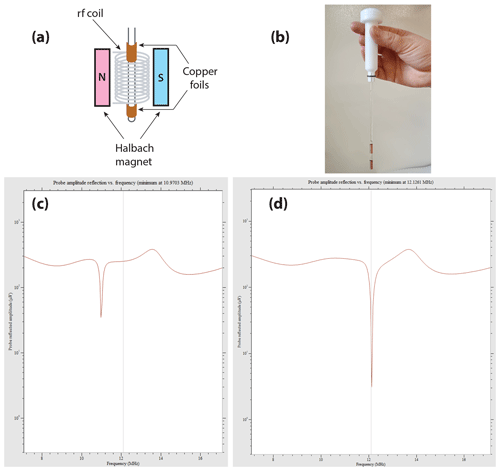Figure 10Example of the effect observed on the wobble curve of the X channel on the Magritek Spinsolve Carbon 43 when simple copper foils are wrapped around the NMR tube. (a) Scheme of the setup, with the copper foils wrapped around the NMR tube; (b) picture of one of these tubes. As we were limited to an outer diameter of 5 mm, the lower part of the NMR tube receiving the foils was only 4 mm; (c) wobble curve of the X channel without the copper foils; (d) wobble curve with the copper coils.

Working with a spectrometer not tuned to the frequency of interest was only possible as (i) it is a low field and (ii) the lowering of the magnet temperature could improve the situation. However, some concern may still appear when using a long rf saturation in the 129Xe UFZ experiments, and every solution to bring the radio frequency of the X channel of the spectrometer closer to the resonance frequency of the nuclei of interest is appealing. An easy and low-cost solution was found by placing a solenoid around the NMR tube or even better by wrapping two copper foils around the NMR tube on either side of the region to be detected, as displayed in Fig. 10a–b, a setup inspired by . The resulting inductive tuning decreases the inductance and thereby induces a raise in the resonance frequency of the circuit.

Figure 10c–d show the wobble curves obtained with the naked NMR tube (c) and with copper foils manually adjusted on the NMR tube axis (d). Remarkably, the quality factor of the probe is not degraded by the presence of the copper foils, and a better matching is even obtained. At the 129Xe frequency, the radiofrequency reflected amplitude at the probe which is ca. 35 µV without the foils (Fig. 10c) falls to 3 µV with them (Fig. 10d).

As can be seen in Fig. 10b, given the gap between the two copper foils, which should be smaller than the original detection zone, a partial rf shielding of the sample must occur. This decreases the detection volume accordingly.

Note also that such a simple setup gives access to all nuclei in the region 10.9–12.1 MHz, i.e., 13C, 27Al, 23Na and 129Xe. It is a priori useable on every benchtop spectrometer, as they are commonly based on a Halbach magnet for the static magnetic field and a solenoid for the rf coil, whose principal axis is colinear to the NMR tube and the magnet aperture. Creating a inductive coupling with this arrangement is therefore an easy task.

Code availability

The software code for the data conversion from Spinsolve format to JCAMP-DX format used in this paper is available from the authors on request.

Data availability

The raw NMR data sets and the GNUPLOT scripts are available for download from https://mycore.core-cloud.net/index.php/s/df2PLypVoITwznn ​​​​​​​.

Supplement

Author contributions

PB conceived and designed the experiments. KC had the idea and developed the coils to shift the working frequency of the benchtop spectrometer. EL, PB and CB performed the NMR experiments at high and low magnetic fields. HD and PB wrote the simulation programs. PB and EL processed the data. PB wrote the manuscript, which was reviewed by all the authors.

Competing interests

The authors declare that they have no conflict of interest.

Special issue statement

Acknowledgements

The authors warmly thank Thierry Brotin (ENS Lyon, France) for providing samples of cryptophanes, Jean-Claude Berthet (CEA Saclay, France) for the preparation of the SEOP cells, and Craig Eccles (Magritek, Germany) for his help on the SpinSolve Expert software.

Financial support

This research has been supported by the Agence Nationale de la Recherche (grant nos. ANR-19-CE19-0024 PHOENIX and ANR-17-LCV2-0002 DESIR).

Review statement

This paper was edited by Fabien Ferrage and reviewed by Ville-Veikko Telkki and Alexej Jerschow.

References

Ahola, S., Zhivonitko, V. V., Mankinen, O., Zhang, G., Kantola, A. M., Chen, H.-Y., Hilty, C., Koptyug, I. V., and Telkki, V.-V.: Ultrafast multidimensional Laplace NMR for a rapid and sensitive chemical analysis, Nat. Commun., 6, 8363, https://doi.org/10.1038/ncomms9363, 2015. a

Berthault, P. and Boutin, C.: Biosensing and Study of Biological Cells using Hyperpolarized 129Xe, chap. 14, in: New Developments in NMR, edited by: Meersmann, T. and Brunner, E, Royal Society of Chemistry, Cambridge, 261–271, https://doi.org/10.1039/9781782628378-00261, 2015. a

Berthault, P., Bogaert-Buchmann, A., Desvaux, H., Huber, G., and Boulard, Y.: Sensitivity and Multiplexing Capabilities of MRI Based on Polarized 129Xe Biosensors, J. Am. Chem. Soc., 130, 16456–16457, https://doi.org/10.1021/ja805274u, 2008. a

Berthault, P., Huber, G., and Desvaux, H.: Biosensing using laser-polarized xenon NMR/MRI, Prog. Nucl. Mag. Res. Sp., 55, 35–60, https://doi.org/10.1016/j.pnmrs.2008.11.003, 2009. a

Bodet, O., Goerke, S., Behl, N. G. R., Roeloffs, V., Zaiss, M., and Bachert, P.: Amide proton transfer of carnosine in aqueous solution studied in vitro by WEX and CEST experiments: Study of Amide Proton Transfer in Carnosine-Water System, NMR Biomed., 28, 1097–1103, https://doi.org/10.1002/nbm.3343, 2015. a

Boutin, C., Stopin, A., Lenda, F., Brotin, T., Dutasta, J.-P., Jamin, N., Sanson, A., Boulard, Y., Leteurtre, F., Huber, G., Bogaert-Buchmann, A., Tassali, N., Desvaux, H., Carrière, M., and Berthault, P.: Cell uptake of a biosensor detected by hyperpolarized 129Xe NMR: The transferrin case, Bioorgan. Med. Chem., 19, 4135–4143, https://doi.org/10.1016/j.bmc.2011.05.002, 2011. a

Boutin, C., Léonce, E., Brotin, T., Jerschow, A., and Berthault, P.: Ultrafast Z-Spectroscopy for 129Xe NMR-Based Sensors, J. Phys. Chem. Lett., 4, 4172–4176, https://doi.org/10.1021/jz402261h, 2013. a, b

Brotin, T. and Dutasta, J.-P.: Cryptophanes and Their Complexes – Present and Future, Chem. Rev., 109, 88–130, https://doi.org/10.1021/cr0680437, 2009. a

Cai, K., Haris, M., Singh, A., Kogan, F., Greenberg, J. H., Hariharan, H., Detre, J. A., and Reddy, R.: Magnetic resonance imaging of glutamate, Nat. Med., 18, 302–306, https://doi.org/10.1038/nm.2615, 2012. a

Cates, G. D., Schaefer, S. R., and Happer, W.: Relaxation of spins due to field inhomogeneities in gaseous samples at low magnetic fields and low pressures, Phys. Rev. A, 37, 2877–2885, https://doi.org/10.1103/PhysRevA.37.2877, 1988. a

Chambers, J. M., Hill, P. A., Aaron, J. A., Han, Z., Christianson, D. W., Kuzma, N. N., and Dmochowski, I. J.: Cryptophane Xenon-129 Nuclear Magnetic Resonance Biosensors Targeting Human Carbonic Anhydrase, J. Am. Chem. Soc., 131, 563–569, https://doi.org/10.1021/ja806092w, 2009. a

Chauvin, C., Liagre, L., Boutin, C., Mari, E., Léonce, E., Carret, G., Coltrinari, B., and Berthault, P.: Note: Spin-exchange optical pumping in a van, Rev. Sci. Instrum., 87, 016105, https://doi.org/10.1063/1.4940928, 2016. a, b

Chighine, K., Léonce, E., Boutin, C., Desvaux, H., and Berthault, P.: Raw NMR data and Gnuplot scripts, MyCore, available at: https://mycore.core-cloud.net/index.php/s/df2PLypVoITwznn, last access: 11 June 2021. a

Desvaux, H. and Berthault, P.: Study of dynamic processes in liquids using off-resonance rf irradiation, Prog. Nucl. Mag. Res. Sp., 35, 295–340, https://doi.org/10.1016/S0079-6565(99)00011-4, 1999. a

Döpfert, J., Witte, C., and Schröder, L.: Fast Gradient-Encoded CEST Spectroscopy of Hyperpolarized Xenon, ChemPhysChem, 15, 261–264, https://doi.org/10.1002/cphc.201300888, 2014a. a

Döpfert, J., Zaiss, M., Witte, C., and Schröder, L.: Ultrafast CEST imaging, J. Magn. Reson., 243, 47–53, https://doi.org/10.1016/j.jmr.2014.03.008, 2014b. a

Driehuys, B., Cofer, G. P., Pollaro, J., Mackel, J. B., Hedlund, L. W., and Johnson, G. A.: Imaging alveolar-capillary gas transfer using hyperpolarized 129Xe MRI, P. Natl. Acad. Sci. USA, 103, 18278–18283, https://doi.org/10.1073/pnas.0608458103, 2006. a

Dubost, E., Dognon, J.-P., Rousseau, B., Milanole, G., Dugave, C., Boulard, Y., Léonce, E., Boutin, C., and Berthault, P.: Understanding a Host-Guest Model System through 129Xe NMR Spectroscopic Experiments and Theoretical Studies, Angew. Chem. Int. Ed., 53, 9837–9840, https://doi.org/10.1002/anie.201405349, 2014. a

Gouilleux, B., Farjon, J., and Giraudeau, P.: Gradient-based pulse sequences for benchtop NMR spectroscopy, J. Magn. Reson., 319, 106810, https://doi.org/10.1016/j.jmr.2020.106810, 2020. a

Guo, Q., Zeng, Q., Jiang, W., Zhang, X., Luo, Q., Zhang, X., Bouchard, L.-S., Liu, M., and Zhou, X.: A Molecular Imaging Approach to Mercury Sensing Based on Hyperpolarized 129Xe Molecular Clamp Probe, Chem. Eur. J., 22, 3967–3970, https://doi.org/10.1002/chem.201600193, 2016. a

Hane, F. T., Li, T., Smylie, P., Pellizzari, R. M., Plata, J. A., DeBoef, B., and Albert, M. S.: In vivo detection of cucurbituril, a hyperpolarized xenon contrast agent for a xenon magnetic resonance imaging biosensor, Sci. Rep., 7, 41027, https://doi.org/10.1038/srep41027, 2017. a

Huber, G., Brotin, T., Dubois, L., Desvaux, H., Dutasta, J.-P., and Berthault, P.: Water Soluble Cryptophanes Showing Unprecedented Affinity for Xenon: Candidates as NMR-Based Biosensors, J. Am. Chem. Soc., 128, 6239–6246, https://doi.org/10.1021/ja060266r, 2006. a, b

Jeong, K., Slack, C. C., Vassiliou, C. C., Dao, P., Gomes, M. D., Kennedy, D. J., Truxal, A. E., Sperling, L. J., Francis, M. B., Wemmer, D. E., and Pines, A.: Investigation of DOTA-Metal Chelation Effects on the Chemical Shift of 129Xe, ChemPhysChem, 16, 3573–3577, https://doi.org/10.1002/cphc.201500806, 2015. a

Khan, N. S., Riggle, B. A., Seward, G. K., Bai, Y., and Dmochowski, I. J.: Cryptophane-Folate Biosensor for 129Xe NMR, Bioconjugate Chem., 26, 101–109, https://doi.org/10.1021/bc5005526, 2015. a

King, J. N., Lee, V. J., Ahola, S., Telkki, V.-V., and Meldrum, T.: Ultrafast Multidimensional Laplace NMR Using a Single-Sided Magnet, Angew. Chem. Int. Ed., 55, 5040–5043, https://doi.org/10.1002/anie.201511859, 2016. a

King, J. N., Fallorina, A., Yu, J., Zhang, G., Telkki, V.-V., Hilty, C., and Meldrum, T.: Probing molecular dynamics with hyperpolarized ultrafast Laplace NMR using a low-field, single-sided magnet, Chem. Sci., 9, 6143–6149, https://doi.org/10.1039/C8SC01329B, 2018. a

Korchak, S., Kilian, W., Schröder, L., and Mitschang, L.: Design and comparison of exchange spectroscopy approaches to cryptophane–xenon host–guest kinetics, J. Magn. Reson., 265, 139–145, https://doi.org/10.1016/j.jmr.2016.02.005, 2016. a

Kunth, M., Witte, C., and Schröder, L.: Quantitative chemical exchange saturation transfer with hyperpolarized nuclei (qHyper-CEST): Sensing xenon-host exchange dynamics and binding affinities by NMR, J. Chem. Phys., 141, 194202, https://doi.org/10.1063/1.4901429, 2014. a

Kunth, M., Witte, C., and Schröder, L.: Continuous-wave saturation considerations for efficient xenon depolarization: Saturation Pulse Considerations for Efficient Xenon Depolarization, NMR Biomed., 28, 601–606, https://doi.org/10.1002/nbm.3307, 2015. a

Léonce, E., Dognon, J.-P., Pitrat, D., Mulatier, J.-C., Brotin, T., and Berthault, P.: Accurate pH Sensing using Hyperpolarized 129Xe NMR Spectroscopy, Chem. Eur. J., 24, 6534–6537, https://doi.org/10.1002/chem.201800900, 2018. a, b

Liu, Z., Dimitrov, I. E., Lenkinski, R. E., Hajibeigi, A., and Vinogradov, E.: UCEPR: Ultrafast localized CEST-spectroscopy with PRESS in phantoms and in vivo: Ultrafast Localized CEST-Spectroscopy with PRESS, Magn. Reson. Med., 75, 1875–1885, https://doi.org/10.1002/mrm.25780, 2016. a

Milani, J., Vuichoud, B., Bornet, A., Miéville, P., Mottier, R., Jannin, S., and Bodenhausen, G.: A magnetic tunnel to shelter hyperpolarized fluids, Rev. Sci. Instrum., 86, 024101, https://doi.org/10.1063/1.4908196, 2015. a

Milanole, G., Gao, B., Paoletti, A., Pieters, G., Dugave, C., Deutsch, E., Rivera, S., Law, F., Perfettini, J.-L., Mari, E., Léonce, E., Boutin, C., Berthault, P., Volland, H., Fenaille, F., Brotin, T., and Rousseau, B.: Bimodal fluorescence/129Xe NMR probe for molecular imaging and biological inhibition of EGFR in Non-Small Cell Lung Cancer, Bioorgan. Med. Chem., 25, 6653–6660, https://doi.org/10.1016/j.bmc.2017.11.002, 2017. a

Riggle, B. A., Greenberg, M. L., Wang, Y., Wissner, R. F., Zemerov, S. D., Petersson, E. J., and Dmochowski, I. J.: A cryptophane-based “turn-on” 129Xe NMR biosensor for monitoring calmodulin, Org. Biomol. Chem., 15, 8883–8887, https://doi.org/10.1039/C7OB02391J, 2017. a

Rose, H. M., Witte, C., Rossella, F., Klippel, S., Freund, C., and Schroder, L.: Development of an antibody-based, modular biosensor for 129Xe NMR molecular imaging of cells at nanomolar concentrations, P. Natl. Acad. Sci. USA, 111, 11697–11702, https://doi.org/10.1073/pnas.1406797111, 2014. a

Schilling, F., Schröder, L., Palaniappan, K. K., Zapf, S., Wemmer, D. E., and Pines, A.: MRI Thermometry Based on Encapsulated Hyperpolarized Xenon, Chem. Eur. J. Chem. Phys., 11, 3529–3533, https://doi.org/10.1002/cphc.201000507, 2010. a

Schnurr, M., Volk, I., Nikolenko, H., Winkler, L., Dathe, M., and Schröder, L.: Functionalized Lipopeptide Micelles as Highly Efficient NMR Depolarization Seed Points for Targeted Cell Labelling in Xenon MRI, Adv. Biosys., 4, 1900251, https://doi.org/10.1002/adbi.201900251, 2020. a

Schröder, L., Lowery, T. J., Hilty, C., Wemmer, D. E., and Pines, A.: Molecular Imaging Using a Targeted Magnetic Resonance Hyperpolarized Biosensor, Science, 314, 446–449, https://doi.org/10.1126/science.1131847, 2006. a

Schröder, L., Meldrum, T., Smith, M., Lowery, T. J., Wemmer, D. E., and Pines, A.: Temperature Response of Xe 129 Depolarization Transfer and Its Application for Ultrasensitive NMR Detection, Phys. Rev. Lett., 100, 257603, https://doi.org/10.1103/PhysRevLett.100.257603, 2008. a

Spence, M. M., Rubin, S. M., Dimitrov, I. E., Ruiz, E. J., Wemmer, D. E., Pines, A., Yao, S. Q., Tian, F., and Schultz, P. G.: Functionalized xenon as a biosensor, P. Natl. Acad. Sci. USA, 98, 10654–10657, https://doi.org/10.1073/pnas.191368398, 2001. a

Taratula, O., Bai, Y., D'Antonio, E. L., and Dmochowski, I. J.: Enantiopure cryptophane- 129Xe nuclear magnetic resonance biosensors targeting carbonic anhydrase, Supramol. Chem., 27, 65–71, https://doi.org/10.1080/10610278.2014.906601, 2015. a

Tassali, N., Kotera, N., Boutin, C., Léonce, E., Boulard, Y., Rousseau, B., Dubost, E., Taran, F., Brotin, T., Dutasta, J.-P., and Berthault, P.: Smart Detection of Toxic Metal Ions, Pb2+ and Cd2+, Using a 129Xe NMR-Based Sensor, Anal. Chem., 86, 1783–1788, https://doi.org/10.1021/ac403669p, 2014. a

Truxal, A. E., Cao, L., Isaacs, L., Wemmer, D. E., and Pines, A.: Directly Functionalized Cucurbituril as a Biosensor for the Selective Detection of Protein Interactions by 129Xe hyperCEST NMR, Chem. Eur. J., 25, 6108–6112, https://doi.org/10.1002/chem.201900610, 2019. a

Vinogradov, E., Sherry, A. D., and Lenkinski, R. E.: CEST: From basic principles to applications, challenges and opportunities, J. Magn. Reson., 229, 155–172, https://doi.org/10.1016/j.jmr.2012.11.024, 2013. a

Walker, T. G. and Happer, W.: Spin-exchange optical pumping of noble-gas nuclei, Rev. Mod. Phys., 69, 629–642, https://doi.org/10.1103/RevModPhys.69.629, 1997. a

Wei, Q., Seward, G. K., Hill, P. A., Patton, B., Dimitrov, I. E., Kuzma, N. N., and Dmochowski, I. J.: Designing 129Xe NMR Biosensors for Matrix Metalloproteinase Detection, J. Am. Chem. Soc., 128, 13274–13283, https://doi.org/10.1021/ja0640501, 2006. a

Wheeler, D. D. and Conradi, M. S.: Practical exercises for learning to construct NMR/MRI probe circuits, Concepts Magn. Reson., 40A, 1–13, https://doi.org/10.1002/cmr.a.21221, 2012. a

Xu, X., Lee, J.-S., and Jerschow, A.: Ultrafast Scanning of Exchangeable Sites by NMR Spectroscopy, Angew. Chem. Int. Ed., 52, 8281–8284, https://doi.org/10.1002/anie.201303255, 2013.  a

Xu, X., Yadav, N. N., Song, X., McMahon, M. T., Jerschow, A., van Zijl, P. C., and Xu, J.: Screening CEST contrast agents using ultrafast CEST imaging, J. Magn. Reson., 265, 224–229, https://doi.org/10.1016/j.jmr.2016.02.015, 2016. a

Yang, S., Jiang, W., Ren, L., Yuan, Y., Zhang, B., Luo, Q., Guo, Q., Bouchard, L.-S., Liu, M., and Zhou, X.: Biothiol Xenon MRI Sensor Based on Thiol-Addition Reaction, Anal. Chem., 88, 5835–5840, https://doi.org/10.1021/acs.analchem.6b00403, 2016. a

Yang, S., Yuan, Y., Jiang, W., Ren, L., Deng, H., Bouchard, L. S., Zhou, X., and Liu, M.: Hyperpolarized 129Xe Magnetic Resonance Imaging Sensor for H 2 S, Chem. Eur. J., 23, 7648–7652, https://doi.org/10.1002/chem.201605768, 2017. a

Zaiss, M., Schnurr, M., and Bachert, P.: Analytical solution for the depolarization of hyperpolarized nuclei by chemical exchange saturation transfer between free and encapsulated xenon (HyperCEST), J. Chem. Phys., 136, 144106, https://doi.org/10.1063/1.3701178, 2012. a, b